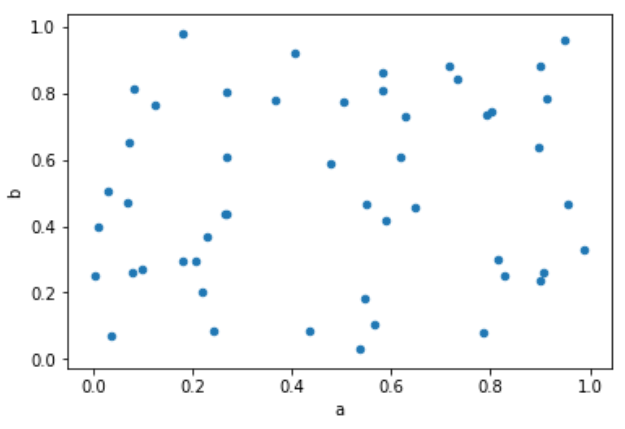Wechat: yu389741| Email: gisdqy@163.com

# (28)Python散点图## 绘制散点图

``````import pandas as pd
import numpy as np
df = pd.DataFrame(np.random.rand(50, 4), columns=['a', 'b', 'c', 'd'])
df.plot.scatter(x='a', y='b')
```Python```